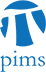## Number Theory Seminar: Explicit modular approaches to generalized Fermat equations

• Date: 04/15/2010
Lecturer(s):
David Brown (UC Berkeley)
Location:

University of British Columbia

Description:

Let $a,b,c \geq 2$ be integers satisfying $1/a + 1/b + 1/c > 1$. Darmon and Granville proved that the generalized Fermat equation $x^a + y^b = z^c$ has only finitely many coprime integer solutions; conjecturally something stronger is true: for $a,b,c \geq 3$ there are no non-trivial solutions and for $(a,b,c) = (2,3,n)$ with $n \geq 10$ the only solutions are the trivial solutions and $(\pm 3,-2,1)$ (or $(\pm 3,-2,\pm 1)$ when n is even).

I'll explain how the modular method used to prove Fermat's last theorem adapts to generalized Fermat equations and use it to solve the equation $x^2 + y^3 = z^{10}$. One new ingredient is the use of number field enumeration techniques to classify Galois representations associated to hypothetical solutions; classically one uses Ribet's level lowering theorem, but here the representations are wildly ramified and his method does not apply.

Schedule:

4:00 - 4:50pm, WMAX 110.

Refreshments will be served before the talk.